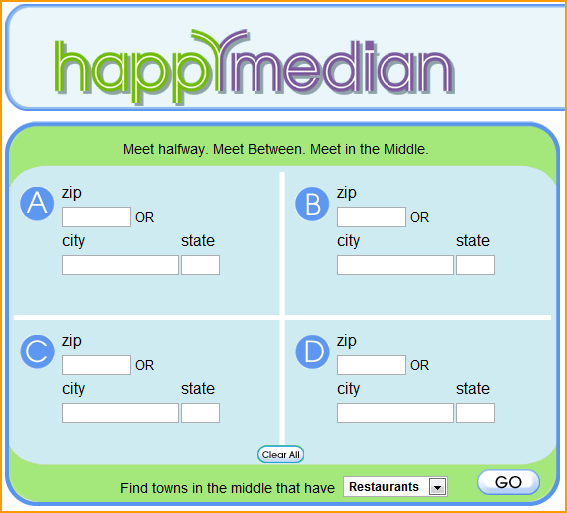# median calculation tool

4195
9
05-03-2016 11:02 AMNew Contributor II

Currently working on a Median calculation tool. This tool is similar to the one I posted on a previous post involving selecting a city and zooming into it. In this case, we have to type in a country name, where the tool will then select all the cities in that country, sort the POP_RANK of the cities in that country, and calculate the median POP_RANK for that country. I was able to complete the select and zoom aspect but I am not entirely sure how to create a list/search cursor which will allow us to input a calculation. Is there a specific way I should approach this?  Any and all help is appreciated!! Thank you

import arcpy

from arcpy import mapping

#sets the workspace

mxd = arcpy.mapping.MapDocument("CURRENT")

df = arcpy.mapping.ListDataFrames(mxd, "Layers")

fc = ("N:/data2016/Lab13/cities.shp")

cities = arcpy.mapping.ListLayers(mxd, "Cities")

cities.showLabels = True

def countryList():

country = arcpy.GetParameterAsText(0)

country_Layer = arcpy.MakeFeatureLayer_management(fc, "country_lyr")

arcpy.SelectLayerByAttribute_management(cities, "NEW_SELECTION", "CNTRY_NAME = '{}' ".format(country))

arcpy.mapping.ListDataFrames(mxd).zoomToSelectedFeatures()

arcpy.RefreshActiveView()

arcpy.Delete_management("country_lyr")

Tags (4)
9 RepliesbyMVP Esteemed Contributor

SearchCursor—Help | ArcGIS for Desktop example 2 shows how to ge the unique values.. if you leave out the set(...) bit, you have all the values, which then you can then use a python sort, get the len(gth) and if len is divisible by 2 (mod), just take the average of the middle 2 (from len/2 and len/2 +1) otherwise if len is odd, just take the middle one.

alternately that field can be read and converted to a numpy array for which there are median methods for data with and without nodata values.byMVP Esteemed Contributor

forgot the code link http://www.arcgis.com/home/item.html?id=6c384f06c9f14d09920f4ff14460f4e2

and your chance to join a cause to free statistical tools Free Frequency ... join the causebyMVP Esteemed Contributor

If you install ArcGIS Pro, you will be using python 3.4 which comes with the Statistics module, which has 4 variants of the median which covers most useable situations 9.7. statistics — Mathematical statistics functions — Python 3.4.4 documentationbyMVP Esteemed Contributor

Does anyone in the 'global reach' section have any further ideas? or should I mark this 'assumed answered'byMVP Frequent Contributor

There was a median calculation tool posted here somewhere by Caleb Mackey.

But no joy with search. So here it is again.

`'''Written By Caleb Mackey4/17/2013Calculates Median Statistics'''import arcpy, os, sys, traceback# env settingsarcpy.env.overwriteOutput = Truearcpy.env.qualifiedFieldNames = Falsedef GetMedian(in_list):    sorted_list = sorted(in_list)    median = int(round(len(sorted_list) / 2))    if len(sorted_list)%2==0:        med_val = float(sorted_list[median-1]                        + sorted_list[median]) / 2    else:        med_val = sorted_list[median]    return med_valdef GetMedianValues(source_fc, new_table, case_field, value_field):        ''' Generates a table with Median Values, summarized by case_field. If the        goal is to get the median for the entire table, use a case field that has        the same value for all records.        source_fc - input feature class to compute median statistics for        new_table - output table        case_field - similar to dissolve field, computes stats based on unique values in this field        value_field - field that contains the actual values for statistics; must be numeric    '''        # Get unique value list for query    print 'starting cursor'    with arcpy.da.SearchCursor(source_fc, [case_field]) as rows:        un_vals = list(set(r for r in rows))    lyr = arcpy.MakeFeatureLayer_management(source_fc,'source_layer')    values = {}    # Get Median UseValue for each station name    for st in un_vals:        query = '"{0}" = \'{1}\''.format(case_field, st)        arcpy.SelectLayerByAttribute_management(lyr, 'NEW_SELECTION', query)        use_vals = []        with arcpy.da.SearchCursor(lyr, [value_field]) as rows:            for row in rows:                if row != None:                    use_vals.append(row)        if len(use_vals) > 0:            median = GetMedian(use_vals)            values[st] = [median, len(use_vals)]    # Create new Summary Statistics table with median    #    if arcpy.Exists(new_table):        arcpy.Delete_management(new_table)    arcpy.CreateTable_management(os.path.split(new_table),os.path.basename(new_table))    # Get field names and types    for field in arcpy.ListFields(source_fc):        if field.name in [case_field, value_field]:            ftype = field.type            name = field.name            length = field.length            pres = field.precision            scale = field.scale            if name == value_field:                if new_table.endswith('.dbf'):                    name = 'MED_' + value_field[:6]                else:                    name = 'MED_' + value_field                value_field2 = name            arcpy.AddField_management(new_table,name,ftype,pres,scale,length)                # Add frequency field    arcpy.AddField_management(new_table,'FREQUENCY','LONG')    # Insert rows    with arcpy.da.InsertCursor(new_table, [case_field, value_field2, 'FREQUENCY']) as rows:        for k,v in sorted(values.iteritems()):            rows.insertRow((k, v, v))                # report results    print 'Created %s' %os.path.basename(new_table)    arcpy.AddMessage('Created %s' %os.path.basename(new_table))    # .dbf's are automatically given a 'Field1' field...Clean this up    try:        if new_table.endswith('.dbf'):            arcpy.DeleteField_management(new_table, 'Field1')    except:        pass    print 'Done'if __name__ == '__main__':##    # testing##    source_fc = r'C:\Testing\Test.gdb\CSR_by_TWP'####    new_table = r'C:\Testing\Test.gdb\Median_CSR' #gdb test##    new_table = r'C:\Testing\Median_CSR.dbf'  #dbf test##    case_field = 'NAME'##    value_field = 'AVE_CSR'    # Script tool params    source_fc = arcpy.GetParameterAsText(0)    new_table = arcpy.GetParameterAsText(1)    case_field = arcpy.GetParameterAsText(2)    value_field = arcpy.GetParameterAsText(3)    GetMedianValues(source_fc, new_table, case_field, value_field)`byMVP Esteemed Contributor

so if using recent versions of python, it would still be easier to use the builtins

>>> import statistics

>>> a = [1,2,3]

>>> statistics.median(a)

2

>>> b = [1,2,3,4]

>>> statistics.median(b)

2.5

>>>

>>> dir(statistics)

['Decimal', 'Fraction', 'StatisticsError', '__all__', '__builtins__', '__cached__', '__doc__', '__file__', '__loader__', '__name__', '__package__', '__spec__', '_check_type', '_counts', '_decimal_to_ratio', '_exact_ratio', '_ss', '_sum', 'collections', 'math', 'mean', 'median', 'median_grouped', 'median_high', 'median_low', 'mode', 'pstdev', 'pvariance', 'stdev', 'variance']

>>>byMVP Frequent Contributor

Of course Dan,

But I am running 10.3.1 (soon moving to 10.4).

Haven't got anywhere near Pro yet. Need to buy a deep blue before I do.byMVP Esteemed Contributor

I have been using python 3.4 since arcmap 10.2... it is a matter of setupbyMVP Esteemed Contributor

I think we have it wrapped up with this one, in case it is a network question